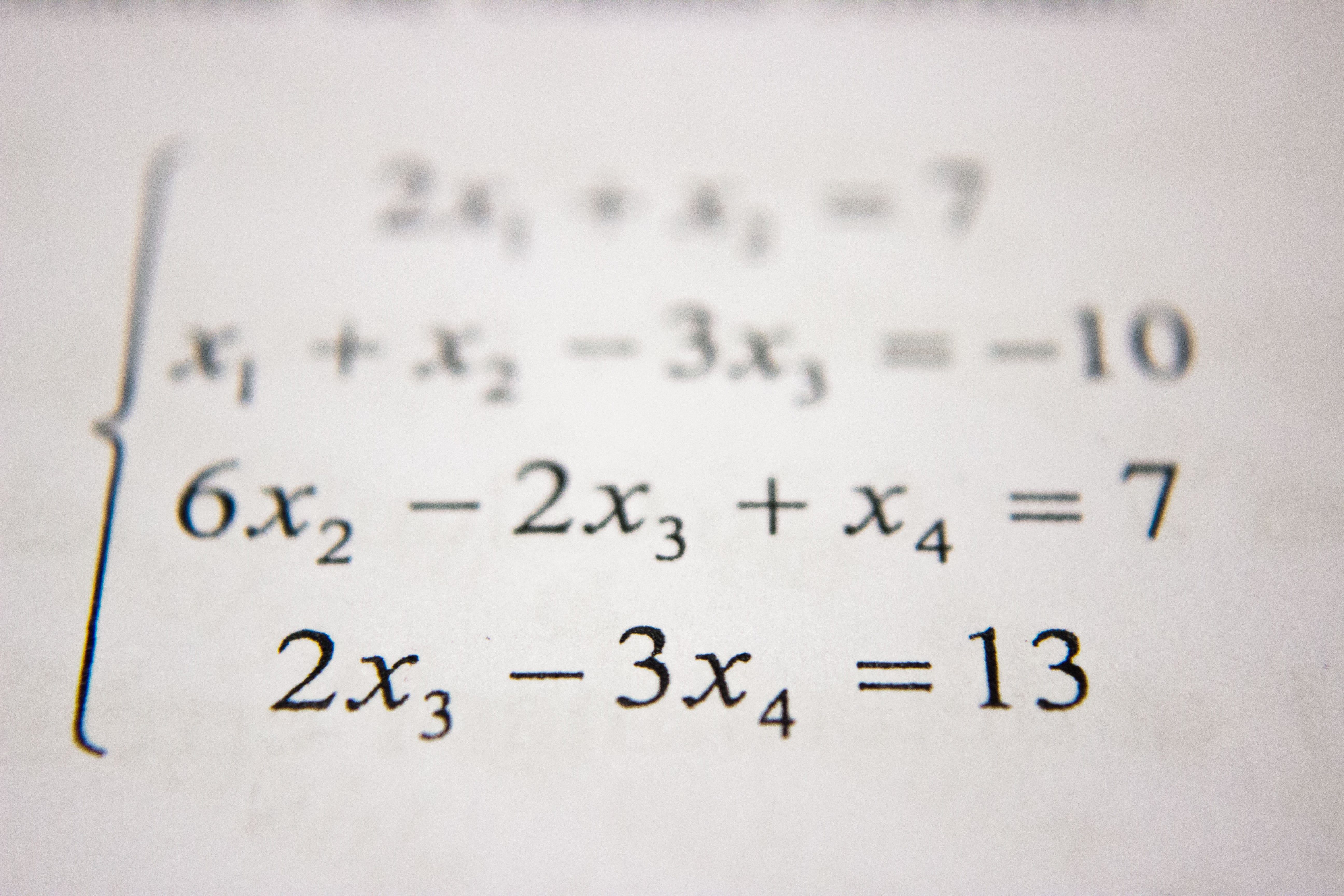# 'Questions threw me off': What you need to know about the 2019 HSC Physics Exam

### There’s a Lot of Maths in the New HSC Physics Exam

The 2019 HSC Physics exam has numbers everywhere. Whether it’s analysing data, performing calculations or rearranging formulae, having a strong foundation in maths will definitely give you an edge in the new physics syllabus.Students planning to study HSC Physics should have a strong foundation of algebra and equations.

8 out of 20 multiple-choice questions and at least 35 out of the 80 marks in the extended response section require either numerical working-out or manipulation of algebraic expressions.

Together, this accounts for at least 47 out of 100 marks. The remaining 53 marks require descriptive responses and examine theory concepts that may still be indirectly related to mathematics.

If you are studying or planning to study physics, hopefully the large proportion of maths is what you expected.

Our recommendations

• Be comfortable with algebra and equations. Focus on these topics throughout Year 9 and 10.
• Study Mathematics at least at the Advanced level (2 Unit)

### Understand the Graphical Relationship Between Variables

Don’t just remember physics formulae and know how to use them to obtain numerical answers. Understand how they can be represented on a graph.

For example, Wien’s Displacement Law is tested in Question 6. Answer for this question is A as the dominant wavelength is inversely proportional to temperature. In other words, the relationship is hyperbolic.Question 31(b) examines Faraday and Lenz’s Law in the form of a student’s hypothesis.Many students would know the cause and effect of back emf, but certainly less would know what the effect would look like on a graph.

Our recommendations:

• Be comfortable with drawing graphs from equations e.g. y=x^2, y=1/x, y=a^x.
• For every new formula you learn, graph every possible relationship. In each graph, ask yourself ‘what is my independent variable, dependent variable and controlled variables?’
• For each graph, think about how you can experimentally validate the graphical relationship.

### Critical Thinking is Important HSC Physics

The last three questions of the 2019 HSC exam took students by surprise. These three questions total up to 20 marks out of the possible 80 in the extended response section.

Students with greater critical thinking ability would have performed better than their peers in these three questions.

Here’s why.

Question 34 is worth 9 marks and combines at least five ideas from both Year 11 and 12 Physics.This question presents students with 4 pieces of data, which can cause confusion and students to feel overwhelmed. As such, a methodical approach is required to make one’s response coherent.

To score high marks in this question, students are required to think on the spot without falling in the trap of categorising the question into one general syllabus dot point. The latter strategy does not work as well in the new HSC Physics exam.

Our recommendations:

• Practise solving problems that involve analysing and interpreting data e.g. graphs and tables
• Don’t limit your preparation to textbooks. Expand the scope of your preparation resources. (See below for our recommendation on resources)
• Practise developing a logical plan before writing long response questions that are worth 7 – 9 marks.

How can I develop critical thinking?

Critical thinking is a problem-solving skill. It is the ability to apply pre-existing knowledge in a new context. Therefore, to improve critical thinking skills, students should practise solving problems in different contexts, formats and styles.

This includes doing non-HSC specific physics questions. This does not mean you need to learn additional concepts but simply practise questions of different styles and format.

For example, the VCE Physics syllabus shares many overlapping concepts with the HSC curriculum, but VCE exam questions are different and therefore can help you developing critical thinking skills.

You can find VCE exam questions sorted by topics that are relevant to the HSC here